﻿ Thermal Resistance Set on Boundary FaceExamples | Product | Murata Software Co., Ltd.# Example15Thermal Resistance Set on Boundary Face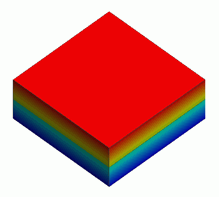### General

• This model consists of two bodies. Their interface has thermal resistivity.

• The temperature distribution and the heat flux vectors are solved.

• Unless specified in the list below, the default conditions will be applied.

### Analysis Space

 Item Settings Analysis Space 3D Model unit mm

### Analysis Conditions

 Item Settings Solver Thermal Analysis [Watt] Analysis Type Steady-state analysis Options N/A

### Model

This model consists two bodies made of aluminum. They share a boundary face.

The thermal resistance is set as a boundary condition on the boundary face. A high temperature is set on the top face of the upper body and a low temperature is set on the bottom face of the other.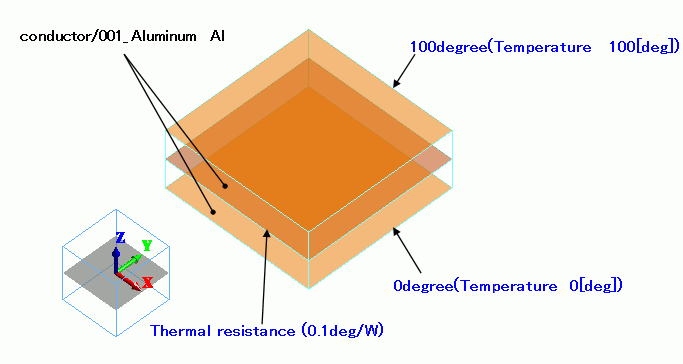### Body Attributes and Materials

 Body Number/Type Body Attribute Name Material Name 0/Solid conductor 001_Al *

* Available from the Material DB

### Boundary Condition

 Boundary Condition Name/Topology Tab Boundary Condition Type Settings 100degree/Face Thermal Temperature 100[deg] 0degree/Face Thermal Temperature 0[deg] TR/Face Thermal Thermal resistance 0.1 [deg/W]

### Results

The temperature distribution is shown below.

The temperature changes discontinuously on paths through the boundary face, on which thermal resistance is set.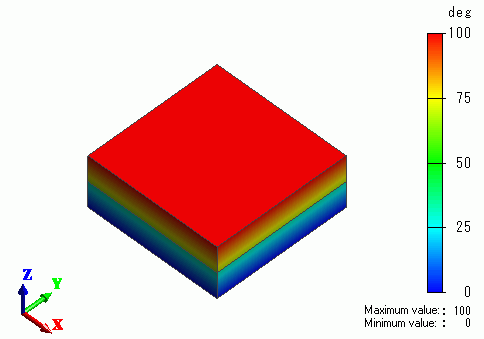The vectors of the heat flux are shown below.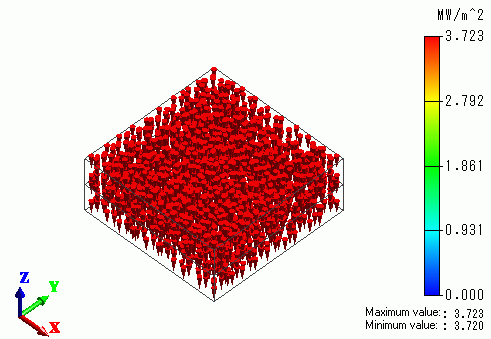The integral of the heat flux is calculated with Integral on Results Window. Calculation result of the heat transfer on the top face of the body is shown below.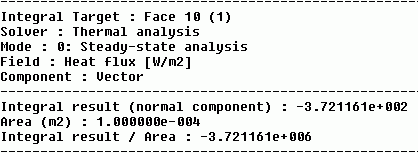Theoretical value is calculated as shown below. Analysis results almost match with the theoretical value.

Thermal resistance of body [deg/W] = Thickness in the heat flux direction [m] / (section area [mm^2] x Thermal conductivity [W/m/deg]),

Body dimensions: 10[mm] x 10[mm] x 2[mm], and Thermal conductivity of aluminium: 237[W/m/deg]

With these, calculation is done as below.

Total thermal resistance = thermal resistance of upper body + thermal resistance at boundary + thermal resistance of lower body

= 2 x 10^-3 / (237 x 100 x 10^-6) + 0.1 + 2 x 10^-3 / (237 x 100 x 10^-6)

= 0.2688 [deg/W]

Overall heat transfer[W] = temperature difference[deg] / total thermal resistance[deg/W]

= (100 – 0 ) / 0.2688

= 372.02 [W]

﻿